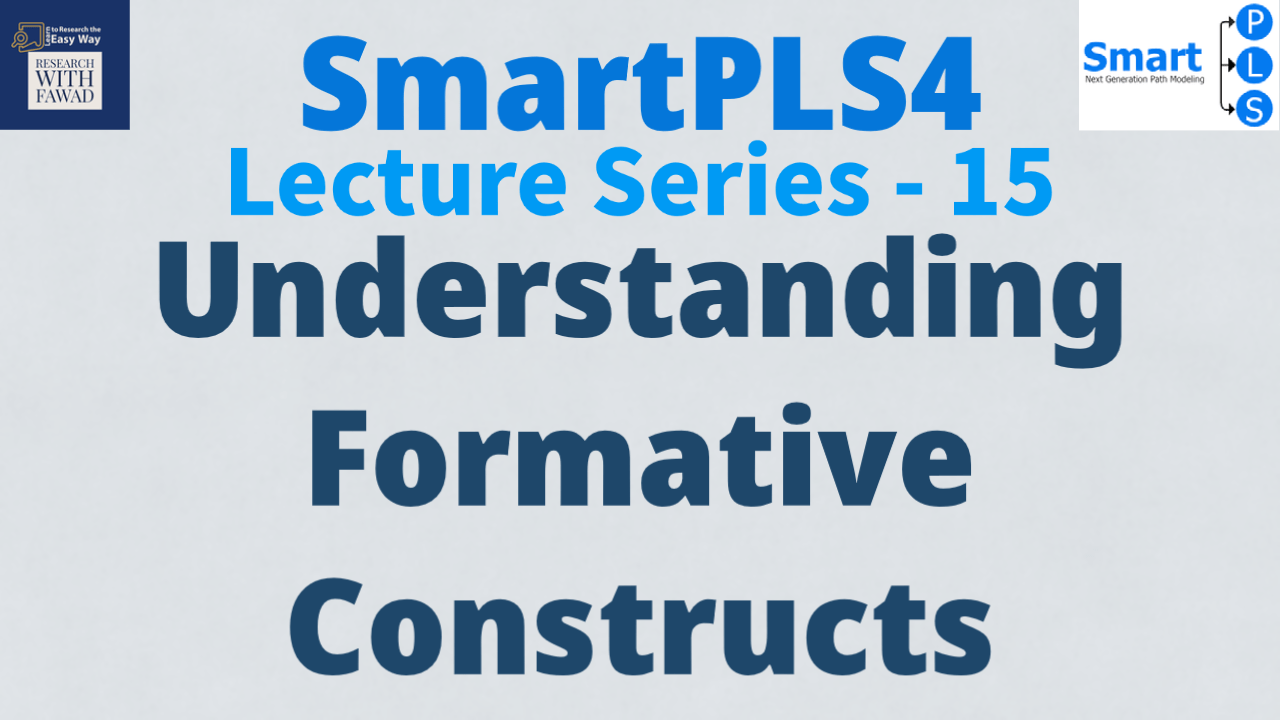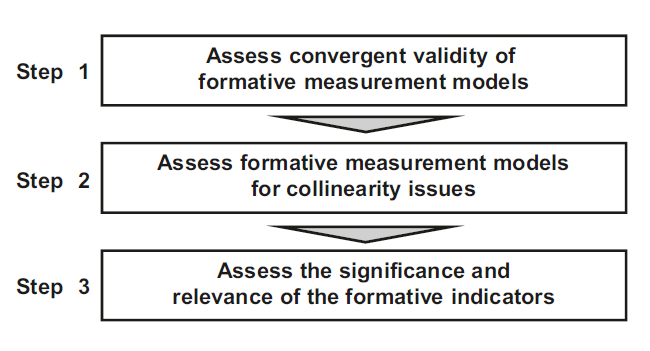# What is a Formative Construct?## Formative Constructs?

• Before assessing the Higher Order Constructs. It is significantly important that the differences between Reflective and Formative Constructs are Discussed.
• The discussion is brief, for a more detail discussion, please visit this video available on the channel (Click Here).

## Formative vs Reflective Constructs

• The relationship between the indicators and the underlying construct can be formative or reflective.
• Latent variables are assessed by observable measures (indicators). The measurement model describes the relationship between these manifest indicators and the latent construct. Measurement models can be reflective or formative in nature.
• In reflective models, the indicators are affected by the latent variable, whereas in formative models the indicators define the latent variable.
• Reflective Indicators are interchangeable since the concept is reflected in the different indicators, sharing a common theme. In a reflective model, the latent construct exists (in an absolute sense) independent of the measures. Since the indicators are used interchangeably, even with the deletion of the one of the indicators,
the latent variable will still exist. whereas Formative indicators are not interchangeable because each indicator contributes a specific meaning to the latent variable.
• In the case of formative models, a change in the indicators results in a change in the construct under study.
• Practically all scales in business and related methodological texts on scale development use a reflective approach to measurement.
• In contrast, in a formative model, the latent construct is dependent upon a constructivist, operationalist or instrumentalist interpretation by the scholar.
• For example, the human development index (HDI) does not exist as an independent entity. Rather, it is a composite measure of human development that includes: health, education and income (UNDP, 2006).
• Any change in one or more of these components is likely to cause a change in a country’s HDI score. In contrast to the reflective model, few examples of formative models are seen in the business literature.
• A reflective measurement theory is based on the idea that latent constructs cause the measured variables and the error results in an inability to fully explain these measures.
• For example, Customer Commitment is believed to cause specific measured indicators like willingness to obtain brand X, telling friends about purchasing brand X, and continuing to buy brand X ay higher price. Here Commitment can be expressed through different ways. Even if one does not tell friends and shows willingness to buy brand X at a higher price, this is still termed Commitment.
• In contrast, a formative measurement theory is modeled based on the assumption that measured variables cause the construct. The error in the formative measurement models is an inability to fulling explain the construct. This means that the indicator list must be comprehensive. For example, the social class index, SCI is a composite of one’s educational level, occupational prestige, and income. SCI does not cause these indicators as in reflective case, but these indicators cause the SCI. In case we remove Income as indicator, we cannot call it SCI.

### How to Validate Formative Constructs?

PLS-SEM is the preferred approach when formatively specified constructs are included in the PLS path model. In this session, I discuss the key steps for evaluating formative measurement models (See Fig). Relevant criteria include the assessment of (1) convergent validity, (2) indicator collinearity, and (3) statistical significance and relevance of the indicator weights.

In the following, key criteria and their thresholds are presented to validate a formative construct.### Step 1: Convergent Validity

In formative measurement model evaluation, convergent validity refers to the degree to which the formatively specified construct correlates with an alternative reflectively measured variable(s) of the same concept. Originally proposed by Chin (1998), the procedure is referred to as redundancy analysis. To execute this procedure for determining convergent validity, researchers must plan ahead in the research design stage by including an alternative measure of the formatively measured construct in their questionnaire. Cheah, Sarstedt, Ringle, Ramayah, and Ting (2018) show that a global single item, which captures the essence of the construct under consideration, is generally sufficient as an alternative measure. Hair et al. (2022) suggest the correlation of the formatively measured construct with the reflectively measured item(s) should be 0.708 or higher, which implies that the construct explains (more than) 50% of the alternative measure’s variance.

### Step 2: Indicator Collinearity

• Collinearity occurs when two or more indicators in a formative measurement model are highly correlated. High correlation increases the standard error of the indicator weights, thereby triggering type II errors (i.e., false negatives). More pronounced levels of collinearity can even trigger sign changes in the indicator weights, which leads to interpretational confounding.
• The standard metric for assessing indicator collinearity is the variance inflation factor (VIF). When VIF values are higher, the level of collinearity is greater. VIF values of 5 or above indicate collinearity problems.
• In this case, researchers should take adequate measures to reduce the collinearity level, for example, by eliminating or merging indicators or establishing a higher-order construct.

### Step 3: Statistical Significance and Relevance of the Indicator Weights

• The third step in assessing formatively measured constructs is examining the statistical significance and relevance (i.e., size) of the indicator weights.
• The indicator weights result from regressing each formatively measured construct on its associated indicators. As such, they represent each indicator’s relative importance for forming the construct. Significance testing of the indicator weights relies on the bootstrapping procedure, which facilitates deriving standard errors from the data
without relying on any distributional assumptions.
• The bootstrapping procedure yields t-values for the indicator weights (and other model parameters). We need to compare these t-values with the critical values from the standard normal distribution to decide whether the coefficients are significantly different from zero. Assuming a significance level of 5%, a t-value above 1.96 (twotailed
test) suggests that the indicator weight is statistically significant. The critical values for significance levels of 1% (α = 0.01) and 10% (α = 0.10) probability of error are 2.576 and 1.645 (two tailed), respectively.
• Confidence intervals are an alternative way to test for the significance of indicator weights. Several types of confidence intervals have been proposed in the context of PLS-SEM. The percentile method is preferred, as it exceeds other methods in terms of coverage and balance, producing comparably narrow confidence intervals. If a confidence interval does not include the value zero, the weight can be considered statistically significant, and the indicator can be retained.
• On the contrary, if the confidence interval of an indicator weight includes zero, this indicates the weight is not statistically significant (assuming the given significance level, e.g., 5%). In such a situation, the indicator should be considered for removal from the measurement model.
• However, if an indicator weight is not significant, it is not necessarily interpreted as evidence of poor measurement model quality. We recommend you also consider the absolute contribution of a formative indicator to the construct, which is determined by the formative indicator’s loading. At a minimum, a formative indicator’s loading should be statistically significant. Indicator loadings of 0.5 and higher suggest the indicator makes a sufficient absolute contribution to forming the construct, even if it lacks a significant relative contribution.

## Videos

### Reference

• Cheah, J. H., Sarstedt, M., Ringle, C. M., Ramayah, T., & Ting, H. (2018). Convergent validity assessment of formatively measured constructs in PLS-SEM. International Journal of Contemporary Hospitality Management, 30(11), 3192–3210.
• Chin, W. W. (1998). The partial least squares approach to structural equation modeling. Modern Methods for Business Research, 295(2), 295–336.
• Hair, J. F., Hult, T., Ringle, C. M., & Sarstedt, M. (2022). A primer on partial least squares structural equation modeling (PLS-SEM) (3rd ed.). Thousand Oaks, CA: Sage.
• Hair Jr, J. F., Hult, G. T. M., Ringle, C. M., Sarstedt, M., Danks, N. P., & Ray, S. (2021). Partial least squares structural equation modeling (PLS-SEM) using R: A workbook.